# Perturbate Normal node

Left Toolbar >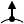Normal Nodes >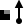Perturbate Normal

The Perturbate Normal node blends two normal images by rotating the normals of the first image by the amount defined by the angles of the second image.

inputsfgbg

outputimage The normals in the baked sphere image get rotated by the normals in the checker image. The effect of the rotation starts at 40 degrees and finishes at 50 degrees.

## Procedure

### To perturbate the normals of two images in Normal Color Mode:

1. On the left toolbar choose Normal Nodes > Perturbate Normal.>2. Drag the Perturbate Normal icon into the Node Graph.3. Connect the output of an image node into the bg** input in the Perturbate Normal node.

4. Connect the output of an image node into the fg input in the Perturbate Normal node.

5. Adjust the attributes of the Perturbate Normal node to modify the blending of the two normal images.

## Inputs

bg
An image in Normal Color Mode.

fg
An image in Normal Color Mode.

An image in Color Color Mode that will be used for masking zones in the input image and preventing them to get modified.

## Outputs

image
An image in Normal Color Mode with final result.

## Attributes PanelMultiply
Multiplies the output angle by the given value, increasing or decreasing the strength of the normal.

Angle Start
Angle on the image input image at which the perturbation starts.

Angle End
Angle on the image input image at which the perturbation ends.

Angle of transition between areas with full and no normal perturbation.

Note: If the Angle Start is greater than the Angle End the perturbation effect is reversed.

## Samples

Perturbate Normal sample 01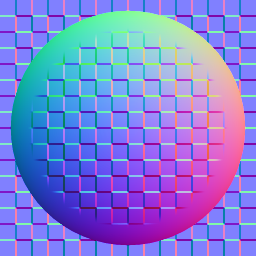Angle Start: 90, Angle End: 60, Angle Fade: 10

Perturbate Normal sample 02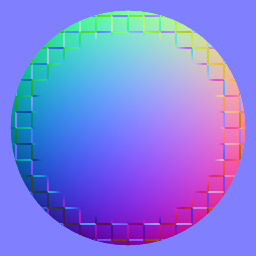Angle Start: 60, Angle End: 90, Angle Fade: 10

Perturbate Normal sample 03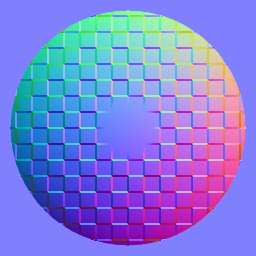Angle Start: 10, Angle End: 00, Angle Fade: 10

Perturbate Normal sample 04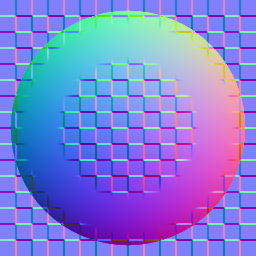Angle Start: 60, Angle End: 40, Angle Fade: 10